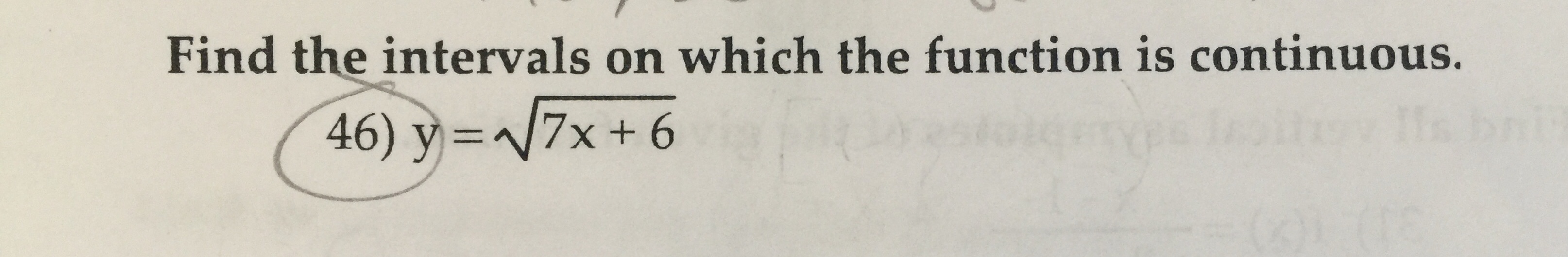# Find the intervals on which the function is continuous.146) y 7x+ 6

Question
3 viewshelp_outlineImage TranscriptioncloseFind the intervals on which the function is continuous. 1 46) y 7x+ 6 fullscreen
check_circle

Step 1

Given a logarithm function:

find the intervals on which the function is continuous

Step 2

Here square root function is given. In order to find the interval on which function is continuous, we will find the domain of the function first.

To find the domain of standard square root function is :

Step 3

Find the domain of gi...

### Want to see the full answer?

See Solution

#### Want to see this answer and more?

Solutions are written by subject experts who are available 24/7. Questions are typically answered within 1 hour.*

See Solution
*Response times may vary by subject and question.
Tagged in

### Continuity